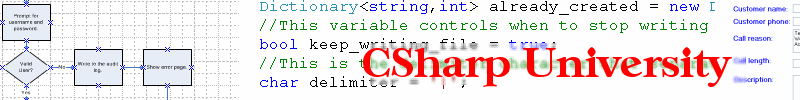"Join my free Amazing ASP.NET newsletter"
It's safe and easy to signup. You get:
• Notified anytime a new article comes out
• Technical step-by-step tutorials on all important ASP.NET topics
• Expert guidance so you can reach unlimited ASP.NET success

Click here to download the sample source code for this if statement lab exercise  If you forgot how to open the files using Visual Web Developer, click here to learn How to open C# ASP.NET Website files.  Please use these files only as a reference.  You should go through the exercises yourself and create your own project according to the instructions in the exercise.

Now that we have covered some basic data types in C#, it’s time to move on to the programming concept of conditionals and loops in .NET.  Conditional statements and operators assist the program in making decisions regarding what logic to perform.  Making decisions is one of the basic building block of all programming languages.  In C# we make decisions using the “if” statement.  The idea being “if a certain condition is true, then execute a block of code”.  Create a new website using Visual Web Developer and call the project Lab2.

Go to the Design view for the file Default.aspx.  Drag a new Button onto the form and change the Text property to say “if by itself”.  Drag a new TextBox to the right of the Button control.  Double click the Button to create the event handler method and put the following code for the button:

```

int i = 33;
int j = 33;

if (i == j)
TextBox1.Text = "The numbers are equal";
```

Notice that we have declared and initialized to integer variables i and j with the same value of 33.  In the “if” statement, we are checking if they are the same by using the equality operator ==.  If the answer is yes and the numbers are the same, then the next line of code following the “if” will be executed.  Please note that it is important to use the double equals == to test for equality.

Drag a another new Button onto the form and change the Text property to say “if with an else”.  Drag a new TextBox to the right of the Button control.  Double click the Button to create the event handler method and put the following code for the button:

```

int i = 103;
int j = 55;

if (i == j)
TextBox2.Text = "The numbers are equal";
else
TextBox2.Text = "The numbers are not equal";
```

This time around, I changed the value of the variables i and j so that they are no longer the same.  Then I added an “else” part to the “if” statement so that the C# runtime engine will now have code to execute if the values of those numbers are not the same.  The runtime engine should execute the “else” statement in this example.  We have now given the .NET engine 2 different blocks of code to execute based on a decision.  Let’s see how we can add even more.

Drag a another new Button onto the form and change the Text property to say “if with else if and else”.  Drag a new TextBox to the right of the Button control.  Double click the Button to create the event handler method and put the following code for the button:

```

int i = 103;
int j = 55;
int k = 103;

if (i == j)
TextBox3.Text = "i and j are equal";
else if (i == k)
TextBox3.Text = "i and k are equal";
else
TextBox3.Text = "i doesn't match any of them";
```

Finally, I now added 3 different code paths into the “if” statement that C# can execute.  The runtime engine always starts at the first “if” to test the condition and make a decision.  If the first condition is NOT true, then it continues to the next condition which is the “else if” portion in this example.  You can have multiple “else if” portions, as many as you need.  If none of the “else if” conditions are true, then the “else” block will be executed.  In this example above, only the “else if” block of code will execute because the variables i and k have identical values.